微積分(偏理工)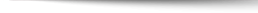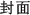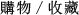Calculus: One and Several Variables 10/e

+作者：

Salas

+年份：
2016 年10 版
+ISBN：
9781119342410
+書號：
MA0460PC
+規格：

+頁數：
1168
+出版商：
John Wiley

\$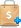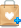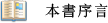More examples, clarifications, and explanatory materials have been added throughout the text as appropriate.Precision and Clarity: The emphasis is on mathematical exposition and topics are treated in a clear and understandable manner.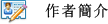Satunino L. Salas is the author of various Wiley calculus textbooks.

Garret Etgen is a professor of mathematics and the University of Houston.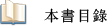Chapter 1. Precalculus Review.
Chapter 2. Limits and Continuity.
Chapter 3. The Derivative; The Process of Differentiation.
Chapter 4. The Mean-Value Theorem; Applications of the First and Second Derivatives.
Chapter 5.  Integration.
Chapter 6.  Some Applications of the Integral.
Chapter 7.  The Transcendental Functions.
Chapter 8.  Techniques of Integration.
Chapter 9.  Some Differential Equations.
Chapter 10.  The Conic Sections; Polar Coordinates; Parametric Equations.
Chapter 11.  Sequences; Indeterminate Forms; Improper Integrals.
Chapter 12.  Infinite Series.
Chapter 13.  Vectors in Three-Dimentional Space.
Chapter 14.  Vector Calculus.
Chapter 15.  Functions of Several Variables.
Chapter 16.  Gradients; Extreme Values; Differentials.
Chapter 17.  Multiple Integrals.
Chapter 18.  Line Integrals and Surface Integrals.
Chapter 19.  Additional Differential Equations.
Appendix A.  Some Additional Topics.
Appendix B.  Some Additional Proofs.